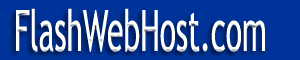# MORSE CODEMorse Code is an International Language. The code was developed by Samuel Morse (1791-1872). All Morse code alphabets are a combination of two elementary characters: a dot (.) and a dash(-). A dot has a length of one time unit and the dash have a length of three units. The length of pause between dot or dash in character is one time unit and length of pause between characters is three units. Similarly length of pause between words equals six time units.

Morse Code is the simplest mode which can be operated upon with simple equipment and can cover large distances that any other mode and therefore will become handy in any emergency situation. Morse code have the ability to override noise and static and still make itself readable. It occupies only a fraction of the bandwidth of radio telephony signals.

Learning morse code is considered as a major stumbing block in passing the examination for a licence. The best way to study morse code is by associationg the sounds with the particular letter. For example the sound dah-di-di-dit is letter B. You can practice morse code by listening to morse code transmissions. I have written a program on C++, which can be used for practicing Morse code. Click here to find more about Morse Tutor.

Below are the morse code characters.

 ```A .- di-dah B -... dah-di-di-dit C -.-. dah-di-dah-dit D -.. dah-di-dit E . dit F ..-. di-di-dah-dit G --. dah-dah-dit H .... di-di-di-dit I .. di-dit J .--- di-dah-dah-dah K -.- dah-di-dah L .-.. di-dah-di-dit M -- dah-dah N -. dah-dit O --- dah-dah-dah P .--. di-dah-dah-dit Q --.- dah-dah-di-dah R .-. di-dah-dit S ... di-di-dit T - dah U ..- di-di-dah V ...- di-di-di-dah W .-- di-dah-dah X -..- dah-di-di-dah Y -.-- dah-di-dah-dah Z --.. dah-dah-di-dit NUMBERS 1 .---- di-dah-dah-dah-dah 2 ..--- di-di-dah-dah-dah 3 ...-- di-di-di-dah-dah 4 ....- di-di-di-di-dah 5 ..... di-di-di-di-dit 6 -.... dah-di-di-di-dit 7 --... dah-dah-di-di-dit 8 ---.. dah-dah-dah-di-dit 9 ----. dah-dah-dah-dah-dit 0 ----- dah-dah-dah-dah-dah ( -.--. dah-di-dah-dah-dit ) -.--.- dah-di-dah-dah-di-dah + .-.-. di-dah-di-dah-dit = -...- dah-di-di-di-dah Full Stop .-.-.- di-dah-di-dah-di-dah [AAA] Comma --..-- dah-dah-di-di-dah-dah [MIM] Semi-colon -.-.-. dah-di-dah-di-dah-dit [NNN] Hyphen -....- dah-di-di-di-di-dah [BA] Question Mark ..--.. di-di-dah-dah-di-dit [IMI] Invitation to transmit -.- dah-di-dah [K] Wait .-... di-dah-di-di-dit [AS] End of Message .-.-. di-dah-di-dah-dit [AR] Error ........ di-di-di-di-di-di-di-dit [HH] Message Received .-. di-dah-dit [R] Sentance Separation Sign -...- dah-di-di-di-dah [BT] ```